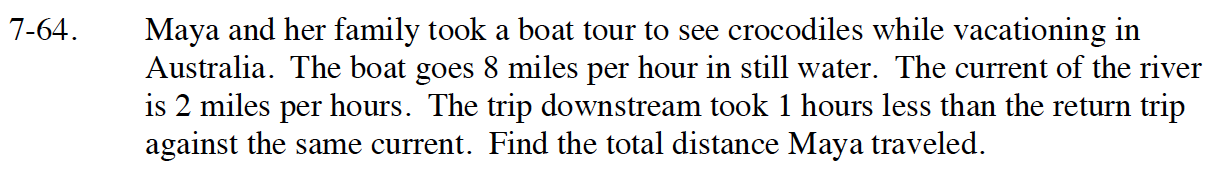Home > CC4 > Chapter 7 > Lesson 7.1.5 > Problem7-64

7-64.

Maya and her family took a boat tour to see crocodiles while vacationing in Australia. The boat goes 8 miles per hour in still water. The current of the river is 2 miles per hours. The trip downstream took 1 hours less than the return trip against the same current. Find the total distance Maya traveled. Homework Help ✎Define your variables; d represents the distance traveled, r represents the rate, and t represents the time to travel upstream.
Also remember d = rt.

d=(8 − 2)t =6 t because t = time traveled upstream and her rate is equivalent to her speed minus the rivers.

d = (8+2)(t − 1)= 10 (t − 1) because time is one hour less, and rate is equivalent to her speed plus the rivers.

What is true about both distances?

So 6t = 10(t − 1), because the distance traveled is the same both ways.

Solve for t and substitute the value back into one of the original distance equations in steps 1 & 2.

30 total miles, because it is 15 miles one way (15 + 15 = 30).